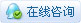2889浏览

# [讨论] [Python]2019网络夏令营之腾讯编程营练习题解答（上）

 本帖最后由 szjuliet 于 2019-10-23 16:37 编辑 2019网络夏令营之腾讯编程营练习题解答（上）（练习中的5个任务） 2019网络夏令营之腾讯编程营练习题解答（下）（练习中另外3个任务） 第十六届深圳市的学生网络夏令营https://coding.qq.com/coding-camp/?Area=shenzhen增加了腾讯编程追梦营的活动适合高中生参加的活动有“编程竞技”示例里有5个小任务。 地址：https://coding.qq.com/coding-lab/?SignUpType=2&CampId=1&Action=Example&DreamId=100 以下是示例的题目及解答，编程的重点不是完成任务，而是引导学生思考解决问题的方法，体会什么是算法。 1. 题目：找最大值 每行有多个数字，找出每行里面的最大数字，并输出其对应的索引值。 输入描述第一行：整数 N， 表示接下来会有 N 行数字接下来的 N 行：每行有多个整数，用逗号分隔 输入例子36,7,8,2,5,6,33,5,3,2,5,3,62,3,5,3,5,8,5 输出描述输出每行最大数字的索引值（每行索引值从 0 开始） ﻿每行输出以换行符 \n 结束。（Python 的 print 语句自带换行符；C/C++ 请使用 "<< endl" 换行） 输出例子265 思路：python有max，min等函数，直接调用就好。当然也可以引导有能力的学生学习排序算法（冒泡、沉底等） [mw_shl_code=python,true]n = int(input()) for i in range(n):     line = input()     numList = list(map(int, line.split(",")))     print(numList.index(max(numList)))[/mw_shl_code]2. 题目：无效的号码 老师收集了所有同学家的电话号码，但是有些粗心的同学可能填错了，把数字填成了字母，或是少填了一些数字。老师让小扣叮帮他找出所有错误的号码。正确的电话号码应该是8位的数字。 输入描述第一行：整数N，表示接下来有 N 行数据接下来的 N 行：每行两个数据，用空格分隔，前面是学生名字，后面是学生的电话号码。 输入例子3A 12345678B 458329b3C 42316 输出描述所有电话号码格式不对的学生名字，每行一个名字。 每行输出以换行符 \n 结束。（Python 的 print 语句自带换行符；C/C++ 请使用 "<< endl" 换行） 输出例子BC 思路：判断字符串的长度、判断字符串是否全是数字 [mw_shl_code=python,true]import sys import math n = int(input()) for i in range(n):     line = input()     name = line.split(" ")     num = line.split(" ")     if len(num) != 8 or not(str.isdigit(num)):         print(name)[/mw_shl_code]3. 题目：装满背包两种物资 A 和 B，单个物资 A 的重量是 3 公斤，单个物资 B 的重量是 7 公斤。 现在有一个能承重 N 公斤 的背包，求解该背包是否可以用 A 和 B 刚好装满（装满是指 N 公斤的背包刚好装了总重为 N 公斤的 A 和 B，不考虑 A 和 B 的形状大小，只考虑重量）。 物资 A 和物资 B 的使用个数不限。 输入描述一个整数 N (3 <= N <= 1000)，表示背包能承受的重量大小（公斤） 输入例子10 输出描述刚好装满的话，输出：YES否则输出：NO 输出例子YES//解释： 10 = 3 + 7 思路：这道题目要求不明确，如是否需要输出所有解，是否需要输出具体的AB组合。在输出例子里要求有解释，但是实际执行时，输出解释程序执行不通过。不过不必纠结细节。这个任务的点还是比较多的，可以引导学生由简单到复杂，逐步完成。算法既可以暴力求解，也可以加上一定的逻辑来简化。 求出一个解：[mw_shl_code=python,true]import sys import math n = int(input()) # 背包的承重 A = 3 #A的重量 B = 7 #B的重量 status = "NO" maxB = int(n) // B #背包可以放B物件的最大个数 s = 0 for i in range(maxB+1):     s = n - i * B     if s % A == 0:         status = "YES"         print(status)         # print("//解释：", n, '=', (s // A) ,'*', A, '+', i, '*', B)         break if status == "NO":     print(status)[/mw_shl_code] 所有测试输出结果都是正确的，但是自动阅卷不通过在IDLE上测试，输出结果完全是正确的。在扣钉平台上测试，输出结果与要求一致，但是运行结果是fail。把“解释”输出注释掉后，运行结果成功。这个与原题要求不符啊。求出所有解： [mw_shl_code=python,true]import sys import math n = int(input()) # 背包的承重 A = 3 #A的重量 B = 7 #B的重量 status = False maxB = int(n) // B #背包可以放B物件的最大个数 s = 0 for i in range(maxB+1):     s = n - i * B     if s % A == 0:         if not(status):             status = True             print("YES")                 print("//解释：", n, '=', (s // A) ,'*', A, '+', i, '*', B)          if not(status):     print("NO")[/mw_shl_code]4. 题目：最小公倍数求出一组数字的最小公倍数 输入描述一行，有 n 个整数，用空格分隔 输入例子3 4 5 输出描述输出这 n 个整数的最小公倍数。 每行输出以换行符 \n 结束。（Python 的 print 语句自带换行符；C/C++ 请使用 "<< endl" 换行） 输出例子60 思路1：先求出n1,n2的最小公倍数n12，再求出n12,n3的最小公倍数n。 [mw_shl_code=python,true]def lcm(a, b):    #  获取最大的数    if a > b:        greater = a    else:        greater = b    while(True):        if((greater % a == 0) and (greater % b == 0)):            lcm = greater            break        greater += 1    return lcm #先求n1、n2的最小公倍数n12，再求n12和n3的最小公倍数n23，依此类推 line = input() numList = list(map(int, line.split(" "))) comm = numList for i in range(len(numList)-1):     comm = lcm(comm,numList[i+1]) #求两数的最小公倍数 print("%d" %comm)[/mw_shl_code]思路2：用两数之积除以两数的最大公约数即为两数的最小公倍数。 [mw_shl_code=python,true]port sys import math #求两数的最大公约数 [mw_shl_code=python,true]import sys import math #求两数的最大公约数 def divisor(a,b):     c = a % b     while c > 0:         a = b         b = c         c = a % b     return b #用最大公约数求最小公倍数 line = input() numList = list(map(int, line.split(" "))) comm = numList for i in range(len(numList)-1):     comm = comm * numList[i+1] / divisor(comm, numList[i+1]) #两数之积除以两数的最大公约数即为两数的最小公倍数 print("%d" %comm)[/mw_shl_code]5. 题目：国王的奖赏小扣叮帮国王解决了一个大问题，国王准备用仓库里的宝贝奖赏小扣叮。仓库是一个 n * n 的方格，有的格子里有宝贝，有的格子是空的。宝贝的价值也有所不同。 奖赏的规则：小扣叮拿了某个宝贝后，同时也可以拿走上下左右四个相邻的格子里的宝贝。 输入描述第一行 1 个整数，表示 n接下来 n 行每行 n 个整数，表示本格子里物品的价值 输入例子50 2 1 0 01 3 0 0 00 0 1 1 00 4 3 1 00 0 0 0 0 输出描述输出一个整数，表示可以获得的最大奖赏。 每行输出以换行符 \n 结束。（Python 的 print 语句自带换行符；C/C++ 请使用 "<< endl" 换行） 输出例子9 分析：这道题相当有趣。题目的重点是算法的实现！引导学生进行头脑风暴，抽丝剥茧找到问题的解决方法。 思路：为了让外围一圈数字能够参与正常循环，我们可以在最外围四周再增加一层数字，它们的值全部为0。 原有是n*n(n=5)的矩阵： 0 2 1 0 01 3 0 0 00 0 1 1 00 4 3 1 00 0 0 0 0 我们将其变换为(n+2)*(n+2)的矩阵，这样原有n*n矩阵中的每一个数字都有上下左右四个数字了： 0 0 0 0 0 0 0 0 0 2 1 0 0 0 0 1 3 0 0 0 0 0 0 0 1 1 0 0 0 0 4 3 1 0 0 0 0 0 0 0 0 00 0 0 0 0 0 0 [mw_shl_code=python,true]import sys import math m = int(input()) + 2 lineList = m * [[]] for i in range(m):     lineList = m *      # print(lineList) max = 0 # print(lineList) for i in range(1,m-1):     line = input()     lineList[1:(m-1)] = list(map(int,(line.split(" ")))) #因为求最大值会用到行为i+1的数值，因此必须全部赋值完成才能进行比较。所以上面的for循环必须单独执行完。 for i in range(1,m-1):     # print(lineList)     for j in range(1,m-1):         s = lineList[i-1][j] + lineList[j-1] + lineList[j] + lineList[j+1] + lineList[i+1][j]         # print(i,j)         # print(lineList[i-1][j] , lineList[j-1] , lineList[j] , lineList[j+1] , lineList[i+1][j])         # print(s)         # print(lineList[i+1][j])         if s > max:             max = s print(max) [/mw_shl_code]您需要登录后才可以回帖 登录 | 立即注册 本版积分规则 回帖并转播 回帖后跳转到最后一页